# Test: Patterns

## 10 Questions MCQ Test Online MCQ Tests for Class 1 | Test: Patterns

Description
Attempt Test: Patterns | 10 questions in 20 minutes | Mock test for Class 1 preparation | Free important questions MCQ to study Online MCQ Tests for Class 1 for Class 1 Exam | Download free PDF with solutions
QUESTION: 1

Solution:
QUESTION: 2

### Choose the figure that is different from the following group.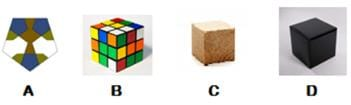Solution:

A is pentagon and all other are Cube.

QUESTION: 3

### What is the shape of the mirror?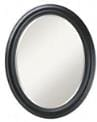Solution:
QUESTION: 4

How many circles are there in pattern given?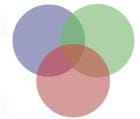Solution:
QUESTION: 5

How many green triangles are there in the given pattern?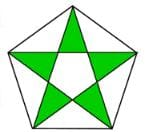Solution:
QUESTION: 6

How many circles are given in the picture?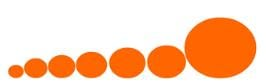Solution:
QUESTION: 7

Identify the number of yellow squares in the picture.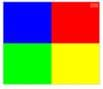Solution:

Only 1 YELLOW square is present.

QUESTION: 8

Identify the odd one out from the following options.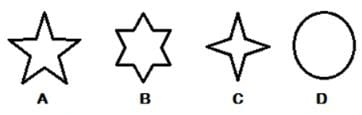Solution:
QUESTION: 9

What are the shapes in the pattern given here?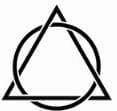Solution:
QUESTION: 10

A  _________ is divided into two halves. Fill in the blanks from the options given below.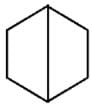Solution:

HexagonUse Code STAYHOME200 and get INR 200 additional OFF Use Coupon Code